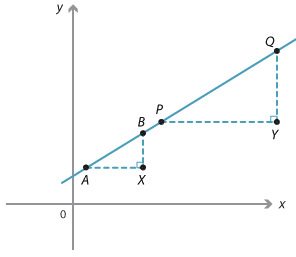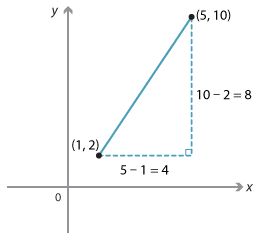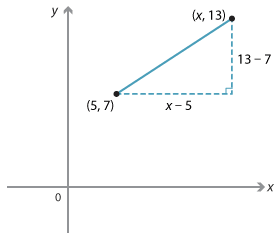Detailed description

The gradient of a line is defined to be the gradient of any interval within the line.
This definition depends on the fact that two intervals on a line have the same gradient.

Suppose AB and PQ are two intervals on the same straight line. Draw right-angled triangles ABX and PQY with sides AX and PY parallel to the x-axis and sides BX and QY parallel to the y-axis.
Triangle ABX is similar to triangle PQY since the corresponding angles are equal. Therefore:

$$\dfrac{QY}{PY} = \dfrac{BX}{AX}$$.

That is, the intervals have the same gradient.

#### Example 6

A line passes through the points (1, 2) and (5, 10). Find its gradient.Detailed description

#### Solution

\begin{align}\text{Gradient} &= \dfrac{y_2 - y_1}{x_2 - x_1}\\\\ &= \dfrac{10 - 2}{5 - 1}\\\\ &= \dfrac{8}{4}\\\\ &=2\end{align}

#### Example 7

A line passes through the point (5, 7) and has gradient $$\dfrac{2}{3}$$. Find the x-coordinate of a point on the line when y = 13.

#### SolutionDetailed description

\begin{align}\text{Gradient of the line} &= \dfrac{6}{x - 5}. \text{Thus:}\\\\ \dfrac{6}{x - 5} &= \dfrac{2}{3}\\\\ 18 &= 2(x\ –\ 5)\\\\ 9 &= x\ –\ 5\\\\ x &= 14\end{align}